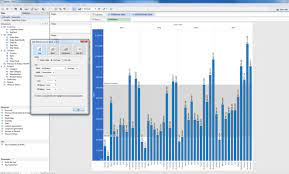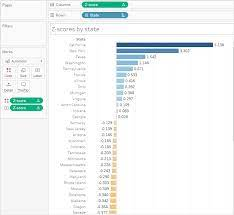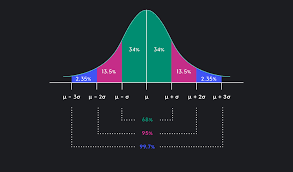6 November 2023

# How to Get Z-Score in Tableau

Understanding and analysing data is a crucial skill for any data professional. Tableau makes visualizing and making sense of data much easier with its highly intuitive and interactive dashboards. One useful technique for working with Tableau is to standardize data using z-scores. But how exactly can you calculate and apply z-scores in Tableau? In this step-by-step tutorial, we'll walk through the process of obtaining z-scores in Tableau. This material is taken from JBI Trainings tableau course

## An Introduction to Tableau and z-Scores

Tableau has become one of the most popular business intelligence and analytics tools used by data analysts and data scientists alike. The data visualization software allows users to transform raw data into impactful interactive charts, graphs, and dashboards.

One of the many benefits of Tableau is its ability to analyze and standardize data through calculations like z-scores. But what exactly is a z-score?

A z-score, also known as a standard score, represents the number of standard deviations a data point is from the mean. It is a statistical measurement that allows you to understand the relationship of an individual data point to the wider dataset.

Z-scores are useful for:

• Normalizing data from different distributions
• Identifying outliers in a dataset
• Comparing data points from different columns/fields
• Understanding if a data point is below, above or aligned with the average

By calculating z-scores in Tableau, data analysts can better visualize, compare and interpret values across multiple data fields. The technique comes in handy when creating interactive Tableau dashboards for data-driven decision making.

Now let's get into the step-by-step process for actually calculating z-scores within Tableau.

## Prerequisites for Calculating z-score

Before diving into the z-score calculation, you need to ensure a few prerequisites are in place:

• Install Tableau Desktop - You'll need to download and install the Tableau Desktop application on your computer. Tableau Online and Public cannot perform calculations.
• Connect to a data source - Connect Tableau to an Excel file, statistical software file, SQL database or any data source containing numeric columns you want to standardize.
• Clean your data - It's good practice to clean your data first by handling blanks, duplicates, formatting issues or any outliers.

Once the data source is connected and prepared, identify the specific columns you want to standardize with the z-score technique. Typically, you'll want to standardize quantitative continuous variables.

## Step 1 - Calculating Statistics

The first step is to calculate the mean and standard deviation required for the z-score formula.

To find the mean:

• Go to the Analytics pane in Tableau Desktop
• Under Statistics, drag `MEAN` onto the Rows shelf
• The mean value for each column will be calculatedTo find the standard deviation:

• Again go to the Analytics pane
• Under Statistics, drag `STDDEV` onto the Rows shelf
• The standard deviation value for each column will be calculated

Take note of the mean and standard deviation for the columns you want to standardize.

## Step 2 - Creating the z-score Calculation

With the mean and standard deviation figures, we can now create the z-score calculation:

The formula for a z-score is:

```z = (x - μ) / σ
```

Where:

• z = z-score
• x = data point value
• μ = mean
• σ = standard deviation

To create this calculation in Tableau:

1. Select the column to standardize
2. Right click and select "Create Calculated Field"
3. Name the new field, e.g. "zscore"
4. Enter the formula:
```( [Value] - [Mean] ) / [Standard Deviation]
```
5. Replace Value, Mean and Standard Deviation with the actual column names
6. Click "OK"

This will create a new zscore calculated field you can now use!The z-score field computes a z-score for each row based on where it falls in relation to the mean and standard deviation of the overall column.

## Step 3 - Applying Z-Score Calculations

With the z-score calculation created, you can now:

• Add it to the view alongside the original data
• Use it to color code marks
• Show reference lines at key z-scores like -1, 0 and 1
• Filter outliers based on z-score thresholds
• Show statistical summaries of the z-scores

Applying z-score analysis allows you to visualize the standardized data for deeper insights.

Here's an example view applying the z-score ranges:By calculating and applying z-scores within Tableau, you can better analyze values across diverse data fields. Use z-scores to identify outliers, patterns and correlations that wouldn't be observable on raw data alone.

## Common Usage Examples of z-scores

Some examples of how z-scores are used by data analysts and scientists include:

• Evaluating outlier data points in statistical analyses
• Comparing values across distinct data distributions
• Identifying under and overperforming metrics in business data
• Segmenting data values into ranges for visualization
• Normalizing data from tests scores, polling results, medical trials etc.
• Determining which datapoints fit into the standard distribution vs exceptional

By calculating z-scores within Tableau, you gain greater insight from visualization dashboards and analytics across functions.

## Limitations and Considerations

While useful, z-scores do come with some limitations and considerations:

• Z-scores can be skewed by outliers in the data
• The mean and standard deviation are influenced by the dataset sample
• Z-scores assume a normal data distribution which may not apply
• Difficult to interpret z-scores if underlying stats are unclear
• Comparing z-scores between different datasets can be misleading

Being aware of these limitations allows you to carefully apply and interpret z-scores within the proper data analytics context.

## Conclusion

Getting a solid handle on z-scores within Tableau opens up deeper statistical insights without complex formulas. By following the steps to calculate and apply z-scores, you can better visualize the relationship between data points. Use this technique to identify outliers, compare values, segment data and reveal hidden patterns.

Now you have the knowledge to start implementing z-score analysis within your Tableau dashboards for more impactful data storytelling.

Here are some common questions around calculating z-scores in Tableau:

Q: Can z-scores be calculated in Tableau Online or Public?

A: No, the analytics and calculated fields required are only available in Tableau Desktop.

Q: What chart types are best for visualizing z-scores?

A: Scatter plots, distribution charts and histograms work well. Color coding marks by z-score ranges also makes patterns clear.

Q: Should I calculate z-scores before importing into Tableau?

A: No need, it's simpler to connect the raw data and then apply calculations like z-score within Tableau.

Q: What are the steps to interpret z-scores in a dashboard?

A: Look at value distributions, filter outliers, identify patterns across marks, observe clustering and gaps.

Q: How are z-scores useful when combined with other Tableau features?

A: Z-scores help in clustering data, highlighting outliers, correlating data sources, segmenting data distributions etc.

If you enjoyed this article check out other articles on our blog. Check out How to Create Top N Parameter in Tableau

If you completed the Tableau course:

If you completed the Tableau Creator - Server / Online course:

If you completed the Tableau Analyst - Beyond the Basics course:

If you completed the R - Reporting & Dashboards with Shiny course:

If you completed the Power BI - Beyond the Basics course:

CONTACT
+44 (0)20 8446 7555

[email protected]

SHAREJB International Training Ltd  -  Company Registration Number: 08458005
Registered Address: Wohl Enterprise Hub, 2B Redbourne Avenue, London, N3 2BS

POPULAR